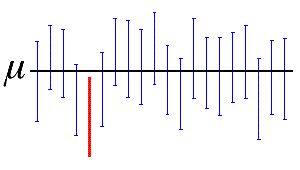# What is a confidence interval?

A confidence interval is a range of values, derived from sample statistics, that is likely to contain the value of an unknown population parameter. Because of their random nature, it is unlikely that two samples from a particular population will yield identical confidence intervals. But if you repeated your sample many times, a certain percentage of the resulting confidence intervals would contain the unknown population parameter.Here, the horizontal black line represents the fixed value of the unknown population mean, µ. The vertical blue confidence intervals that overlap the horizontal line contain the value of the population mean. The red confidence interval that is completely below the horizontal line does not. A 95% confidence interval indicates that 19 out of 20 samples (95%) from the same population will produce confidence intervals that contain the population parameter.

Use the confidence interval to assess the estimate of the population parameter. For example, a manufacturer wants to know if the mean length of the pencils they produce is different than the target length. The manufacturer takes a random sample of pencils and determines that the mean length of the sample is 52 millimeters and the 95% confidence interval is (50,54). Therefore, they can be 95% confident that the mean length of all pencils is between 50 and 54 millimeters.

The confidence interval is determined by calculating a point estimate and then determining its margin of error.
Point Estimate
This single value estimates a population parameter by using your sample data.
Margin of Error

When you use statistics to estimate a value, it's important to remember that no matter how well your study is designed, your estimate is subject to random sampling error. The margin of error quantifies this error and indicates the precision of your estimate.

You probably already understand margin of error as it is related to survey results. For example, a political poll might report that a candidate's approval rating is 55% with a margin of error of 5%. This means that the true approval rating is +/- 5%, and is somewhere between 50% and 60%.

For a two-sided confidence interval, the margin of error is the distance from the estimated statistic to each confidence interval value. When a confidence interval is symmetric, the margin of error is half of the width of the confidence interval. For example, the mean estimated length of a camshaft is 600 mm and the confidence interval ranges from 599 to 601. The margin of error is 1.

The greater the margin of error is, the wider the interval is, and the less certain you can be about the value of the point estimate.

By using this site you agree to the use of cookies for analytics and personalized content.  Read our policy